# Comparison Operators in Google Sheets (>,<,<>,=,>=,<=)

Google Sheets allows us to use more than 6+ comparison operators which can be easily incorporated with the help of IF functions. We can also use comparison operators along with the different functions of Google Sheets such as datasets, filters, conditional formatting operations, comparing columns, and so on.

Let’s understand how to use comparison operators with the help of Google Sheets tips on this page. Read on to find out more.

## What is Comparison Operators in Google Sheets?

Comparison operators compare two values to determine if they are equal, not equal, or whether one is more than and/or equal to the other. Numeric numbers, date and time values, and, in some situations, text values can all be used by comparison operators

The comparison operator simply compares the value on the left-hand side to the value on the right-hand side.

Depending on the results of the comparison, they return a TRUE or FALSE Numerical expression, which can be used to determine whether or not a transformation should be applied to a row, column, or cell.

### Types of Google Sheets Comparison Operators

The types of comparison operators which can be used in Google Sheets are explained below:

 Comparison Operator Name Symbol to be used in Google Sheets Equal to = Not Equal to <> Less than < Greater than > Less than or Equal to <= Greater than or Equal to >=

## Equal To Operator in Google Sheets

The syntax to use the equal to operator is =(value1 = value2).

This operator compares the left and right sides and determines if they are equal. The two values being compared are value1 and value2, which can be numbers, text, strings, date/time values, Boolean values, or pointers to cells having any of these values.

Follow the steps listed below to use the equal to the operator in Google Sheets.

• Step 1: Select the cell where you want to perform this operation.
• Step 2: Now enter the formula as =a2=b2.
• Step 3: If the values are equal, it results as “TRUE“. If the values are not equal, it shows the results “False“.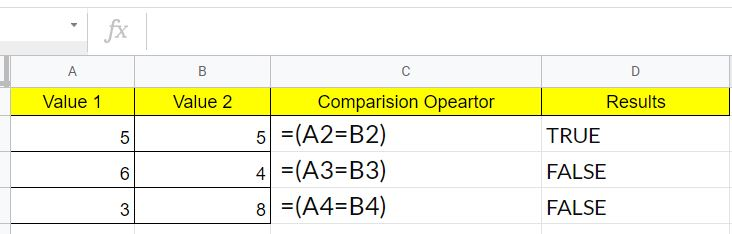From the above example we can see that:

• Since the A2 and B2 have the same value, the operation =(A2=B2) returns TRUE.
• Since the value in A3 is not the same as the value in B3, the operation =(A3=B3) will return the value FALSE.
• For the same reason, the operation =(A4=B4) will return the value FALSE.

### Not Equal To Operator in Google Sheets

The syntax which needs to be used for Not Equal operator is =(value1 <> value2)

This operator compares the left and right sides and determines whether they are not equal. The two values being compared are value1 and value2, which can be numbers, text, strings, date/time values, Boolean values, or pointers to cells having any of these values.

Let’s understand how to use Not Equal to the operator in Google Sheets are given below:

• Step 1: Select the cells where you would like to apply the Not Equal To operator.
• Step 2: Now enter the formula as ” =(A2<>B2)“.
• Step 3: Press the Enter button. It will show the results.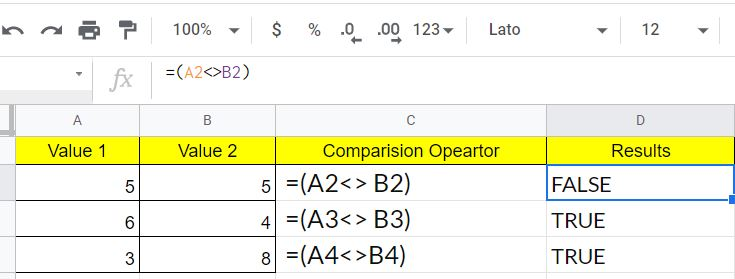Working of Not Equal to Formula

• Since A2 and B2 have the same value, the operation =(A2>B2) returns FALSE.
• Since the value in A3 is not the same as the value in B3, the operation =(A3>B3) returns TRUE.
• The operation =(A4>B4) will return the value TRUE.

### Less Than Operator in Google Sheets

The syntax for using the less-than operator in Google Sheets is =(value1 < value2). This operator compares two values and determines whether the left-hand value is less than the right-hand value. The two values being compared are value1 and value2.

To understand how the Google Sheet ‘Less than’ operator works, consider the following example:

• Step 1: Select the cell where you would like to use the Less Than operator.
• Step 2: Now enter the formula =(A2<B2).
• Step 3: Press the “Enter” key and you will see the results.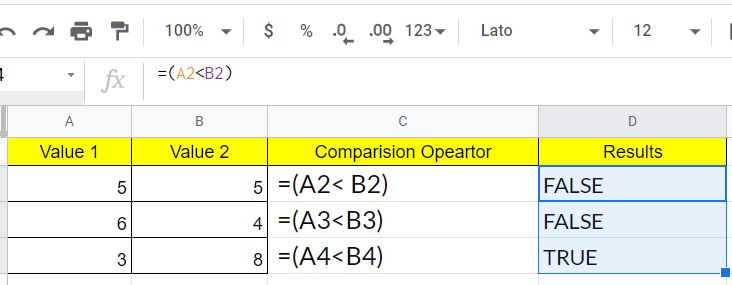Working of Less Than Operator

• Because A2 and B2 have the same value, the operation =(A2<B2) returns FALSE.
• Because the value in A3 is not less than the value in B3, the operation =(A3<B3) will return the value FALSE.
• Similarly, the value in A4 is less than the value in B4, the operation =(A4<B4) returns TRUE.

### Greater Than Operator in Google Sheets

The syntax for using the greater than the operator in Google Sheets are =(value1 > value2). This operator compares two values and determines whether the left-hand value is greater than the right-hand value. The two values being compared are value1 and value2.

To understand how to use the Greater Than Operator in Google Sheets are explained below:

• Step 1: Select the cell where you would like to apply the greater than formula.
• Step 2: Now enter the formula as =(a2>b2).
• Step 3: Press the “Enter“. The value returns True or False based on the comparison.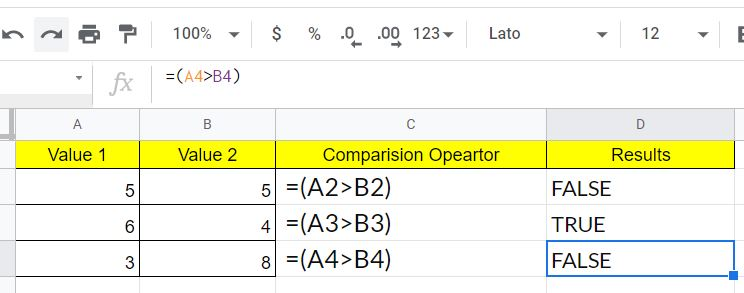Working of Greater Than Function

• The =(A2>B2) operation returns FALSE as A2 has a value equal to B2.
• A value TRUE will then be returned from the operation =(A3>B3) since the A3 value is larger than the B3 value.
• Likewise, the =(A4>B4) operation returns the FALSE value because A3 values are not higher than the B3 value.

### Less Than or Equal To Operator in Google Sheets

The syntax to use the less-than operator in Google Sheets are =(value1 <= value2). This operator compares and checks if the value on the left is less than or equal to the value on the right side.

Steps to Use Less Than or Equal To Operator in Google Sheets

• Step 1: Select the cell where you would like to use the Less than the operator.
• Step 2: Now type the formula =(A2<=B2).
• Step 3: Press the “Enter” button. The results will be displayed on the screen.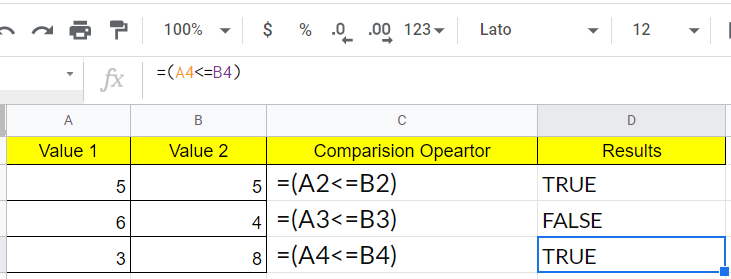Working of Less than or Equal to Operator:

• The value TRUE is returned to the operation =(A2<=B2). The value in A2 is not less than B2, as you can see. The statement is TRUE since A2 is equal to B2.
• The =(A3<=B3) operation returns a FALSE, as the A3 value is equal to or not less than the B3 value.
• The =(A4<=B4) operation will return the value of TRUE as the value of A4 is below that of B4.

### Greater Than or Equal To Operator in Google Sheets

The syntax to use for greater than or equal to operators in Google Sheets are =(value1 >= value2). This operator checks and compares whether the value on the left side is higher or equal to the right side value. Where value1 and value2 are compared to the two values.

Steps to Use Greater Than or Equal To Operator in Google Sheets are:

• Step 1: Select the cell where you would like to use the Greater than or equal to operator.
• Step 2: Now type the formula =(A2>=B2).
• Step 3: Press the “Enter“. The results will be shown on the screen.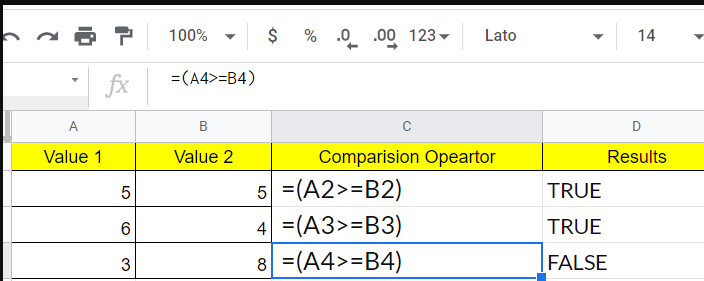Working of Greater than or equal to the operator in Google Sheets

• The value of TRUE will be returned by operation =(A2>=B2). In A2 the value is no higher than B2, as you can see. The statement TRUE however is that A2 is equal to B2.
• The value TRUE will be returned in an operation =(A3>=B3) as it is larger in A3 than in B3.
• Then again, the =(A4>=B4) operation returns the FALSE value, as the A4 values do not exceed or equate to the B4 value.

### Alternative Functions Similar to Comparison Operators in Google Sheets

Apart from the above 6 comparison operators, you can also use 6 comparison functions in Google Sheets as alternatives. They act in the same way that comparison operators do, with the exception of syntax.

 Comparison Operator Name Comparison Operator Symbol Comparison Function Syntax Equal to = EQ EQ(value1,value2) Not Equal to <> NE NE(value1,value2) Less than < LT LT(value1,value2) Greater than > GT GT(value1,value2) Less than or Equal to <= LTE LTE(value1,value2) Greater than or Equal to >= GTE GTE(value1,value2)